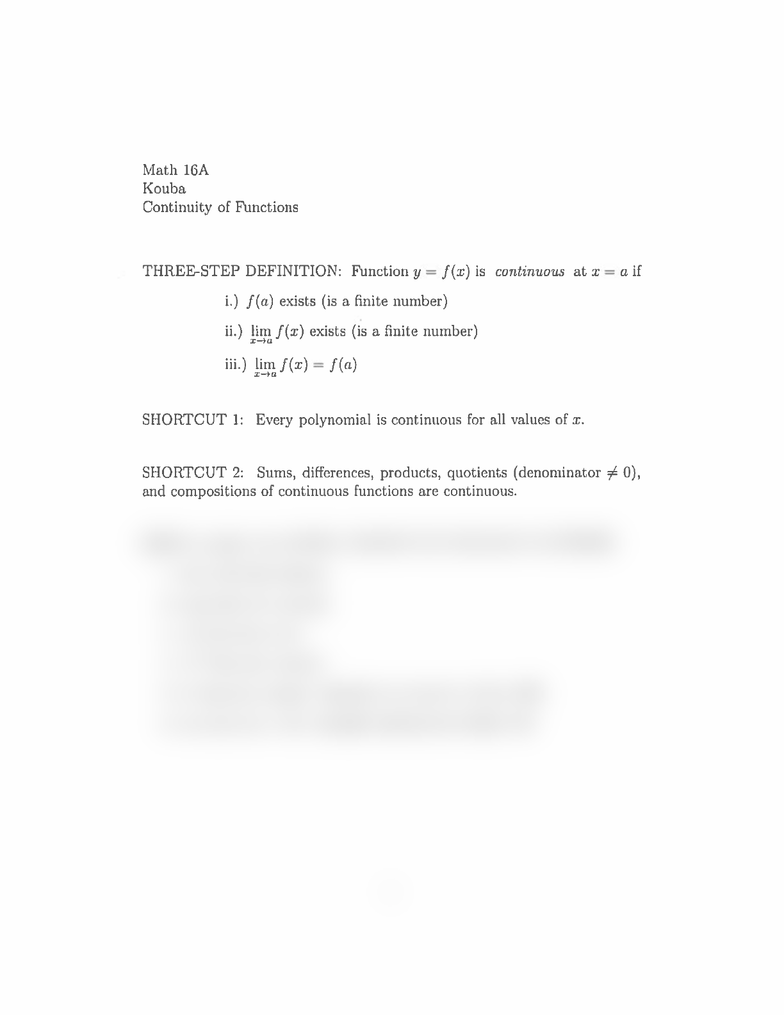Study Guides (390,000)
US (220,000)
UC-Davis (3,000)
MAT (300)
MAT 16A (40)
D.Kouba (10)
Final

# MAT 16A Lecture 14: ContinuityExam

Department
Mathematics
Course Code
MAT 16A
Professor
D.Kouba
Study Guide
Final

This preview shows half of the first page. to view the full 1 pages of the document.Math
16A
Kouba
Continuity
of
Functions
THREE-STEP
DEFINITION:
Function
y
=
f(z)
is
continuous
at
x
=
a
if
i.)
f(a)
exists
(is
a
finite
number)
ii.)
limf(x)
exists
(is
a
finite
number)
Hi.)
Hmf(x)
=
f(a)
SHORTCUT
1:
Every
polynomial
is
continuous
for
all
values
of
x.
SHORTCUT
2:
Sums,
differences,
products, quotients
(denominator
\$
0),
and
compositions
of
continuous
functions
are
continuous.
HERE
is
a
short
list
of
WELL-KNOWN
CONTINUOUS FUNCTIONS:
1.
sinx
(for
all
x-values)
2.
cosx
(for
all
x-values)
3.
/E
(for
all
x
0)
4
‘3
(for
all
x-values)
5.
c
(for
all
x-values)-
formally
introduced
in
Math
16B
6.
lnx
(for
all
x
>
0)
formally
introduced
in
Math
16B
1